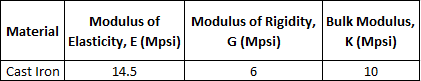## Poisson

Consider a 5 feet long extrusion with a square shaped cross sectional area of 0.5 in^2 is made from Cast Iron. What is the Poisson’s Ratio? Note the properties of Cast Iron in the table shown.Hint
Poisson’s ratio measures a material’s deformation in directions perpendicular to the loading/pressure direction:
$$\upsilon=-\frac{\varepsilon_{lateral}}{\varepsilon_{longitudinal}}$$$where $$\varepsilon_{lateral}$$ is the lateral strain, and $$\varepsilon_{longitudinal}$$ is the longitudinal strain. Hint 2 Bulk (Volume) Modulus of Elasticity: $$K=\frac{E}{3(1-2\nu )}$$$
where $$K$$ is the bulk modulus, $$E$$ is the modulus of elasticity, and $$\nu$$ is Poisson’s ratio.
Poisson’s ratio measures a material’s deformation in directions perpendicular to the loading/pressure direction:
$$\upsilon=-\frac{\varepsilon_{lateral}}{\varepsilon_{longitudinal}}$$$where $$\varepsilon_{lateral}$$ is the lateral strain, and $$\varepsilon_{longitudinal}$$ is the longitudinal strain. Bulk (Volume) Modulus of Elasticity: $$K=\frac{E}{3(1-2\nu )}$$$
where $$K$$ is the bulk modulus, $$E$$ is the modulus of elasticity, and $$\nu$$ is Poisson’s ratio.

Notice how the extrusion dimensions, cross sectional area, and Modulus of Rigidity values can be ignored. They were in the problem statement to cause confusion. Plugging in the modulus of elasticity and bulk modulus values from the Cast Iron properties table:
$$10Mpsi=\frac{14.5Mpsi}{3[1-2(\nu )]}$$$Solving for Poisson’s ratio: $$[1-2(\nu )]=\frac{14.5Mpsi}{(3)10Mpsi}=0.483$$$
$$\nu =\frac{(0.483-1)}{(-2)}=\frac{-0.5167}{-2}=0.26$$\$
0.26